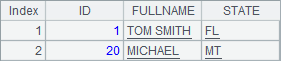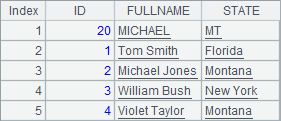# 命令行

## 无返回结果的网格文件

 A B 1 =create(ID,Amount) 2 for 100 >A1.insert(0,#A2,rand(100*100)) 3 =file("D:\\files\\Data1.txt") >A3.export@t(A1)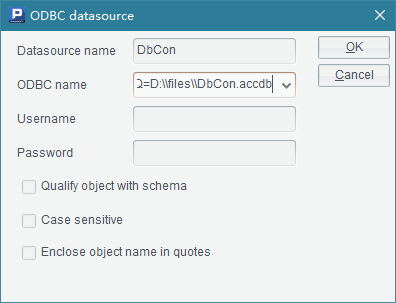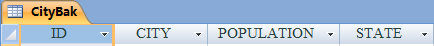A B 1 =file("D:\\files\\Data1.txt") =A1.import@t() 2 for IDs >B1.modify(A2,Value:Amount) 3 >A1.export@t(B1)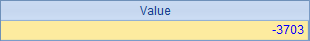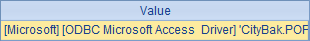## 需要返回结果的网格文件

 A B 1 =days(Day) =string(Day,"EEE") 2 return A1 return B1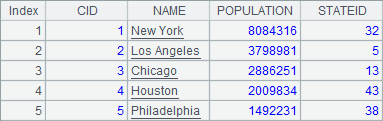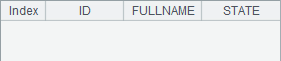<Esproc>

<dateFormat>yyyy-MM-dd </dateFormat>

<timeFormat>HH:mm:ss</timeFormat>

<dateTimeFormat> yyyy-MM-dd HH:mm:ss</dateTimeFormat>

</Esproc>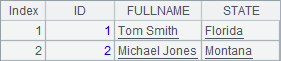A B 1 =days(Day) =string(Day,"EEE") 2 return [A1,B1]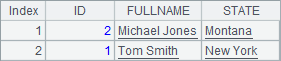A B 1 \$(demo) select * from CITIES \$select * from STATES 2 =A1.switch(STATEID,B1:STATEID) =A2.new(CID,NAME:City,STATEID.NAME:State) 3 =B2.select(left(City,1)==Letter) 4 return A3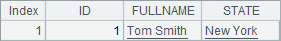<DBList encryptLevel="0">

<DB name="demo">

<property name="url" value="jdbc:hsqldb:hsql://127.0.0.1/demo"/>

<property name="driver" value="org.hsqldb.jdbcDriver"/>

<property name="type" value="13"/>

<property name="user" value="sa"/>

<property name="batchSize" value="1000"/>

<property name="autoConnect" value="true"/>

<property name="useSchema" value="false"/>

</DB>

</DBList >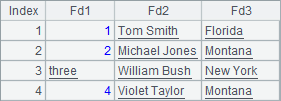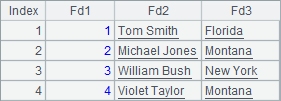## 执行网格脚本和简单 SQL

esprocx命令后添加-c选项，可以执行输入的一个网格脚本，网格脚本中，列用Tab分隔，行用回车分隔，脚本输入完毕后同时按下Ctrl+C结束输入网格并开始执行脚本。如：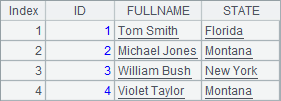-  []  >10.(A1=A1|#)

-  >output(A1)